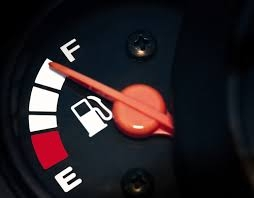# VOCABULARY 16893

delivery. ... . ..9.00 75km/h
At what time and at what distance the van catch up with the truck. WORD PROBLEM ON MOVEMENT.

Result

s =  56.25 km
T = 9:45 hh:mm### Step-by-step explanation:

s = 45•(t-8.5)
s = 75•(t-9.00)

s-45t = -382.5
s-75t = -675

s = 225/4 = 56.25
t = 39/4 = 9.75

Our linear equations calculator calculates it.Did you find an error or inaccuracy? Feel free to write us. Thank you!

Tips for related online calculators
Do you have a linear equation or system of equations and looking for its solution? Or do you have a quadratic equation?
Do you want to convert length units?
Do you want to convert velocity (speed) units?
Do you want to convert time units like minutes to seconds?